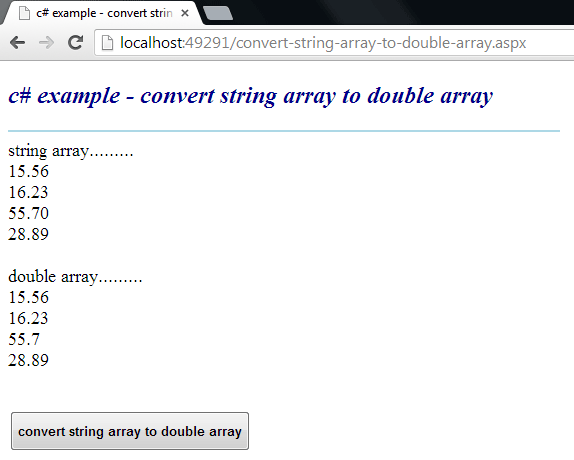### c# - How to convert a string array to a double array

Convert a String Array to a Double Array
The Array class provides methods for creating, manipulating, searching, and sorting arrays. The Array class is not part of the System.Collections namespaces. However, it is still considered a collection because it is based on the IList interface. An element is a value in an Array. The length of an Array is the total number of elements it can contain. The Array has a fixed capacity.

The following .net c# tutorial code demonstrates how we can convert a String Array to a Double Array. That means we will convert a String Array instance whose elements are Double data type to a Double Array object. But there is no direct method to convert a String Array instance to a Double Array object. So, we have to apply some actions to get a Double Array from String Array elements.

In the beginning, we have to initialize an empty instance of Double Array. In the initializing time, we will set the Double Array size the same as the String Array size. Then we will loop through the String Array elements. While iterating the String Array elements, we will set the Double Array elements value corresponding to the String Array elements.

But we have to convert the String value to a Double instance. Here we will use the Convert class ToDouble() method to convert a String instance to a Double instance. So finally, we will get a Double Array instance from the String Array elements.

The Convert class converts a base data type to another base data type. The Convert class ToDouble() method converts a specified value to a double-precision floating-point number.

The Convert class ToDouble(String) method overload converts the specified string representation of a number to an equivalent double-precision floating-point number. The Convert class ToDouble (string? value) method overload has a parameter named value.

The value parameter is a string that contains the number to convert. The Convert class ToDouble(String) method returns a double-precision floating-point number that is equivalent to the number in value, or 0 if the provided value is null.

The Convert class ToDouble (string? value) method overload throws FormatException if the value is not a number in a valid format. It also throws OverflowException if the provided value represents a number that is less than Double.MinValue or greater than Double.MaxValue.
convert-string-array-to-double-array.aspx
``````
<%@ Page Language="C#" AutoEventWireup="true"%>

<!DOCTYPE html>
<script runat="server">
protected void Button1_Click(object sender, System.EventArgs e)
{
string[] stringarray = new string[]
{
"15.56",
"16.23",
"55.70",
"28.89"
};

Label1.Text = "string array.........<br />";
foreach (string s in stringarray)
{
Label1.Text += s + "<br />";
}

double[] darray = new double[stringarray.Length];
for (int i = 0; i < stringarray.Length;i++ )
{
darray[i] = Convert.ToDouble(stringarray[i]);
}

Label1.Text += "<br />double array.........<br />";
foreach (double d in darray)
{
Label1.Text += d.ToString() + "<br />";
}
}
</script>

<html xmlns="http://www.w3.org/1999/xhtml">
<title>c# example - convert string array to double array</title>
<body>
<form id="form1" runat="server">
<div>
<h2 style="color:DarkBlue; font-style:italic;">
c# example - convert string array to double array
</h2>
<hr width="550" align="left" color="LightBlue" />

<asp:Label
ID="Label1"
runat="server"
Font-Size="Large"
>
</asp:Label>
<br /><br />
<asp:Button
ID="Button1"
runat="server"
Text="convert string array to double array"
OnClick="Button1_Click"
Height="40"
Font-Bold="true"
/>
</div>
</form>
</body>
</html>
``````More c# examples